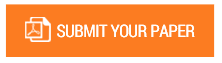On Three Figurate Numbers with Same Values

Authors : A. Vijayasankar, Sharadha Kumar, M. A. Gopalan

Volume/Issue : Volume 4 - 2019, Issue 11 - November

Scribd : https://bit.ly/2rzbYse

Explicit formulas for the ranks of Triangular numbers, Hexagonal numbers, Centered Hexagonal numbers, Centered Octagonal numbers, Centered Decagonal numbers and Centered Dodecagonal numbers satisfying the relations; 3,N 6,h 6,H t  t  ct , t 3,N  t6,h  ct 8,M , t 3,N  t6,h  ct10,M , 3,N 6,h 12,D t  t  ct are obtained.

Keywords : Equality of polygonal numbers, Centered Hexagonal numbers, Centered Octagonal numbers, Centered Decagonal numbers, Centered Dodecagonal numbers, Hexagonal numbers, Triangular numbers.

#### CALL FOR PAPERS

Paper Submission Last Date
31 - May - 2023